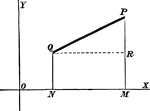### Distance

Showing distance between two points on a coordinate plane.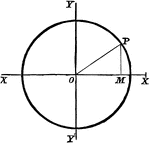### Circle Equation

To find the equation of a circle whose center is the origin and whose radius is 3 units in length.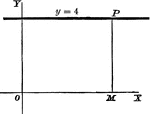### Perpendicular Line

A perpendicular line on a coordinate plane, showing what y the y-coordinate will be.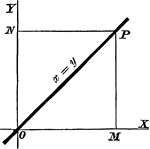### Straight Line

An x=y line on a coordinate plane.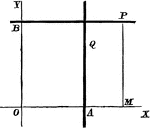### Straight Line

Equation of a straight line: a) parallel to y-axis, b) parallel to x-axis.### Straight Line

A positive slope line making a 60 degree angle with the x-axis.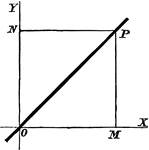### The Origin

A straight line through the origin of a coordinate plane.### Point Ratio

Showing how to find points on a coordinate plane. Also, shows an example of the midpoint formula.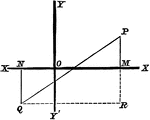### Right Triangle

A right triangle on a coordinate plane.